# Bolt Length Calculation - CADWorx - Help

PPMProduct
PPMCategory_custom
Help
20.1 (2020 R1)

Bolt Type = 0: Fixed Length Bolt

CADWorx does not calculate bolt lengths for type 0 bolts.

Bolt Type = 1: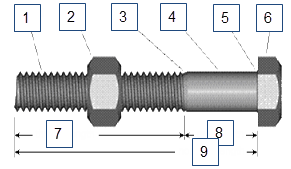1 - Thread 2 - Nut 3 - Runout 4 - Shank 5 - Radius 6 - Head 7 - Thread Length 8 - Grip Length 9 - Nominal Length

Bolt Type = 2: with bolt extension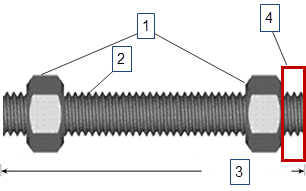1 - Nuts 2 - Thread 3 - Nominal Length 4 - Bolt Extension

Nut Count

Nut count = 1, Washer count = 0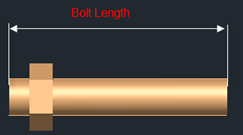Nut count = 2, Washer count = 2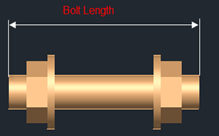Nut count = 5, Washer count = 4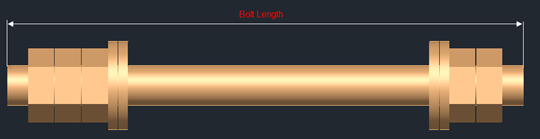Bolt Length Formula

Bolt Type = 1:

BOLT LEN = Round (X + Nut Count * Nut Thickness + Bolt extension + Number Washer * Washer Thickness)

Bolt Type = 2:

BOLT LEN = Round (X + Nut Count * Nut Thickness + 2 * Bolt extension + Number Washer * Washer Thickness)

The bolt length = maximum of (BOLT LEN, Length in the data table).

Where X is: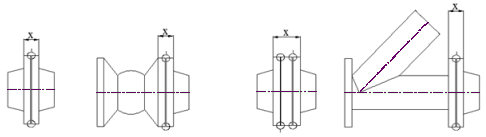The round function works with the BoltRoundControl setting.

BoltRoundControl specifies the rounding of bolt length:

• 0 - No rounding is performed.

• 1 - Rounds the value up to the nearest multiple of the value specified in Tolerance.

• 2 - Rounds the value down to the nearest multiple of the value specified in Tolerance.

• 3 - Rounds the value up or down to the nearest multiple of the value specified in Tolerance.

If the bolt Weight equals 0.0 in the Stud Bolts data table, the software calculates the weight using the Density value from the Material Table and the CAD platform solid volume of the whole set of bolts.

If the bolt Weight is greater than 0.0, then the following applies:

• The software calculates the bolt weight as long as the bolt length calculated in the model is longer than in the data table.

• Bolt weight = (Actual bolt length / length value of the data table)*Weight value of the data table.

Bolt Flanges

Two flange components are required, with optional gaskets and components.

Components in the middle must have a wafer end type on both ends. Two components are allowed.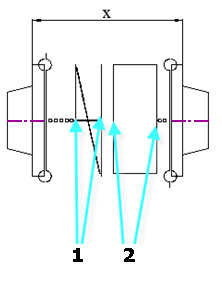1 - Bleed ring 2 - Spectacle blind